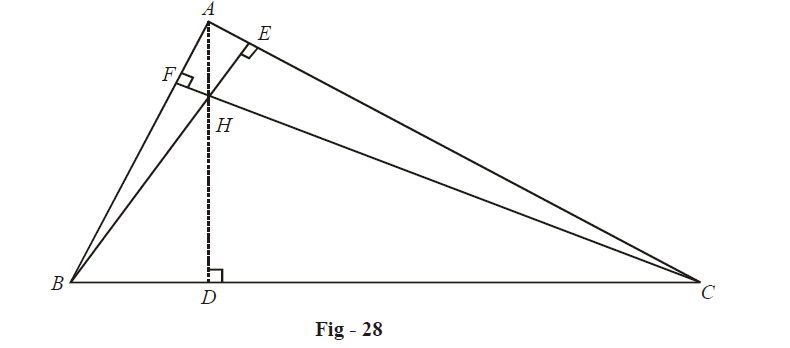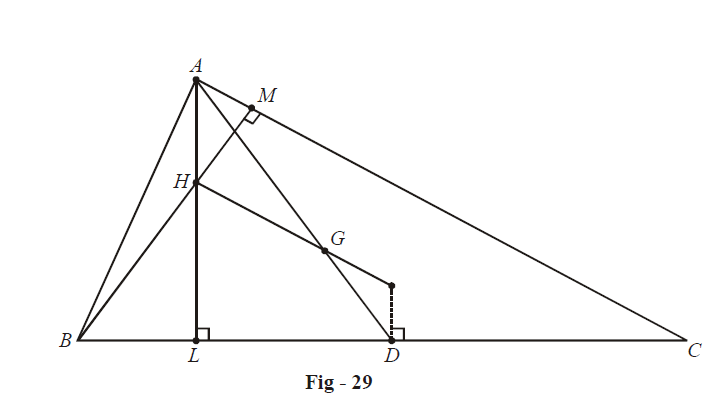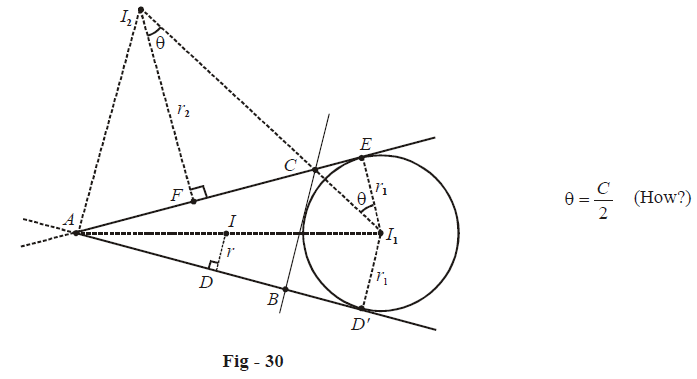# Examples on Circumcircles Incircles and Excircles Set 4

Go back to  'Trigonometry'

Example - 67

Let H be the orthocenter in a triangle ABC as shown:Prove that

(a)  $$HD = 2R\cos B\cos C,\;HE = 2R\cos C\cos A,\;HF = 2R\cos A\cos B$$

(b)  $$HA = 2R\cos A,\;HB = 2R\cos B,\;HC = 2R\cos C$$

Solution: (a) In  $$\Delta BHD$$  ,

\begin{align}&\;\;\;\,\,\,\,\,\,\,\,\,\,\,\,\,\,\,\,\,\tan (\angle HBD) = \frac{{HD}}{{BD}} = \frac{{HD}}{{AB\cos B}} \\ &\Rightarrow \qquad \tan \left( {\frac{\pi }{2} - C} \right) = \frac{{HD}}{{C\cos B}} \\ &\Rightarrow \qquad HD = c\cos B\cot C = \frac{c}{{\sin C}}\cos B\cos C = 2R\cos B\cos C \\ \end{align}

Similarly, we can evaluate expressions for HE and HF

(b) In $$\Delta AEH$$  ,

\begin{align}&\;\;\;\,\,\,\,\,\,\,\,\,\,\,\,\,\,\,\,\cos (\angle HAE) = \frac{{AE}}{{HA}} = \frac{{AB\cos A}}{{HA}} \\ &\Rightarrow \qquad \cos \left( {\frac{\pi }{2} - C} \right) = \frac{{c\cos A}}{{HA}} \\ &\Rightarrow \qquad HA = \frac{{c\cos A}}{{\sin C}} = 2R\cos A \\ \end{align}

We can obtain analogous expressions for HB and HC.

Example - 68

Let G, H, O denote the centroid, orthocenter and circumcentre in $$\Delta ABC$$ . Prove that $$OG:GH = 1:2$$

Solution:Note that  $$OD = R\cos A$$  (how), and using the result of the previous example,  $$HA = 2R\cos A$$  . Thus,

$HA = 2OD$

Also, since G is the centroid,

$AG = 2GD$

Thus, $$\Delta AGH$$ and  $$\Delta DGO$$  are similar with the sides ratio 2 : 1. This implies that  $$OG:GH = 1:2$$ .

Note that we have not proved explicitly that O, G and H are collinear. If this is not evident to you, by all means try to prove it rigorously.

Example - 69

Let  $$I,\;{I_1},\;{I_2},\;{I_3}$$  be the incenter and the three ex-centres of  $$\Delta ABC$$  . Find

(a)  $${I_1}A$$       (b)  $${I_1}A$$

(c)  $$I\;{I_1}$$       (d)   $${I_1}\;{I_2}$$

Solution:(a)  $${\text{In}}\;\Delta AID$$  ,

\begin{align}&\sin \frac{A}{2} = \frac{r}{{AI}} \\\Rightarrow\quad&IA = r\;{\text{cosec}}\frac{A}{2} \\ \end{align}

(b)  $${\text{In}}\;\Delta A{I_1}D',$$

\begin{align}&\sin \frac{A}{2} = \frac{{{r_1}}}{{A{I_1}}} \\\Rightarrow\quad &{I_1}A = {r_1}\;{\text{cosec}}\frac{A}{2} \\\end{align}

(c)  \begin{align}I\,{I_1} = {I_1}A - IA = {r_1}\,{\text{cosec}}\frac{A}{2} - r\;{\text{cosec}}\frac{A}{2}\end{align}

Since \begin{align}{r_1} = s\tan \frac{A}{2}\;{\text{and}}\;r = (s - a)\tan \frac{A}{2},\end{align} we have

$I\,{I_1} = \frac{{s - (s - a)}}{{\cos \frac{A}{2}}} = a\sec \frac{A}{2}$

(d)

\begin{align}&{I_1}\,{I_2} = {I_1}C + C{I_2} = {r_1}\sec \frac{C}{2} + {r_2}\sec \frac{C}{2} \\\\ \,\,\,\,\,\,\,\,\,\, &\qquad\qquad\qquad\quad\;\;= s\left( {\tan \frac{A}{2} + \tan \frac{B}{2}} \right)\sec \frac{C}{2} \\\\ \,\,\,\,\,\,\,\,\,\, &\qquad\qquad\qquad\quad\;\;= s\frac{{\sin \left( {\frac{{A + B}}{2}} \right)\sec \frac{C}{2}}}{{\cos \frac{A}{2}\cos \frac{B}{2}}}\\\\ \,\,\,\,\,\,\,\,\,\,&\qquad\qquad\qquad\quad\;\;= \frac{s}{{\sqrt {\frac{{s(s - a)}}{{bc}}} \sqrt {\frac{{s(s - b)}}{{ca}}} }} \\ \\ \,\,\,\,\,\,\,\,\,\, &\qquad\qquad\qquad\quad\;\;= c\;\;{\text{cosec}}\;\frac{C}{2}\\ \end{align}